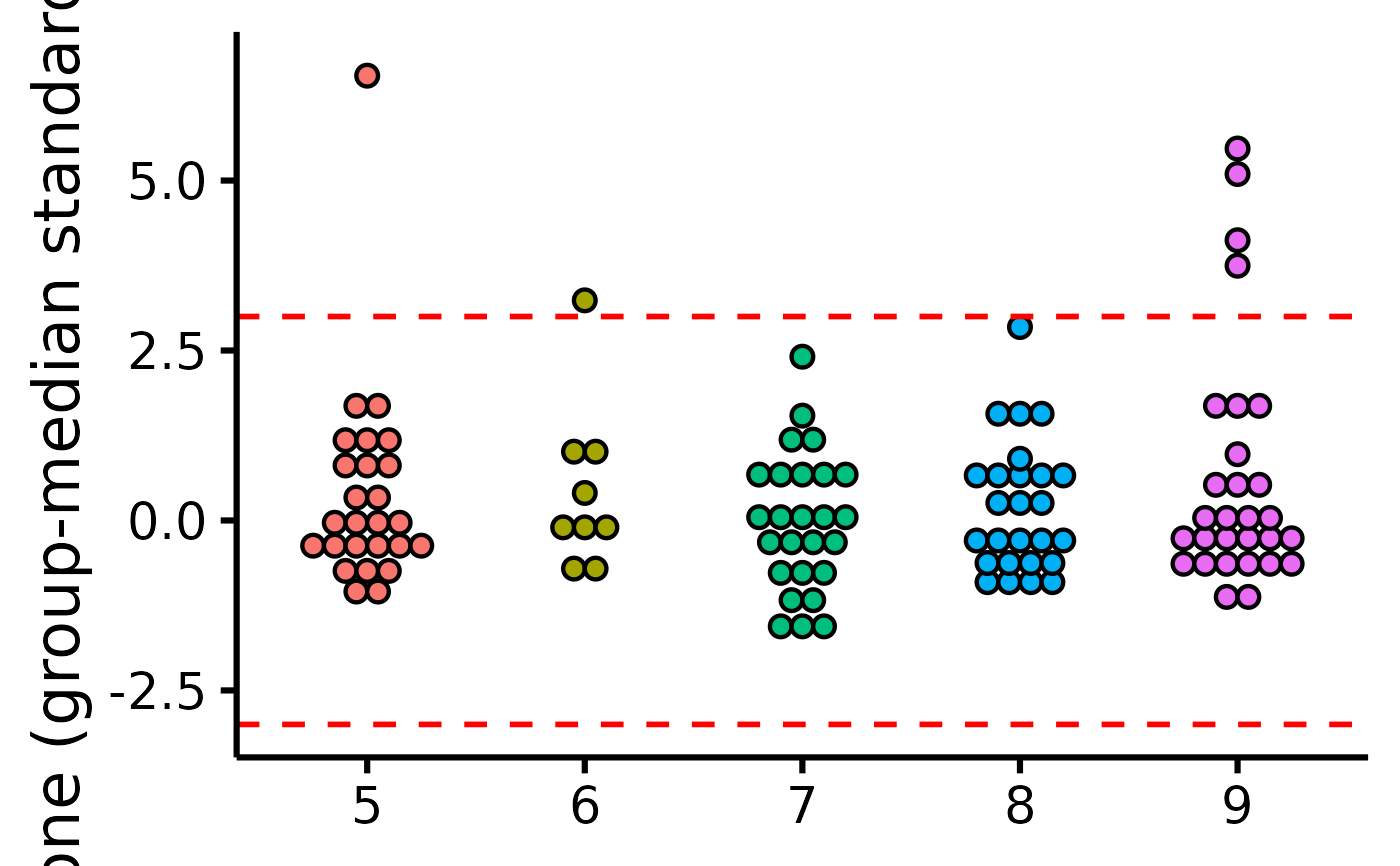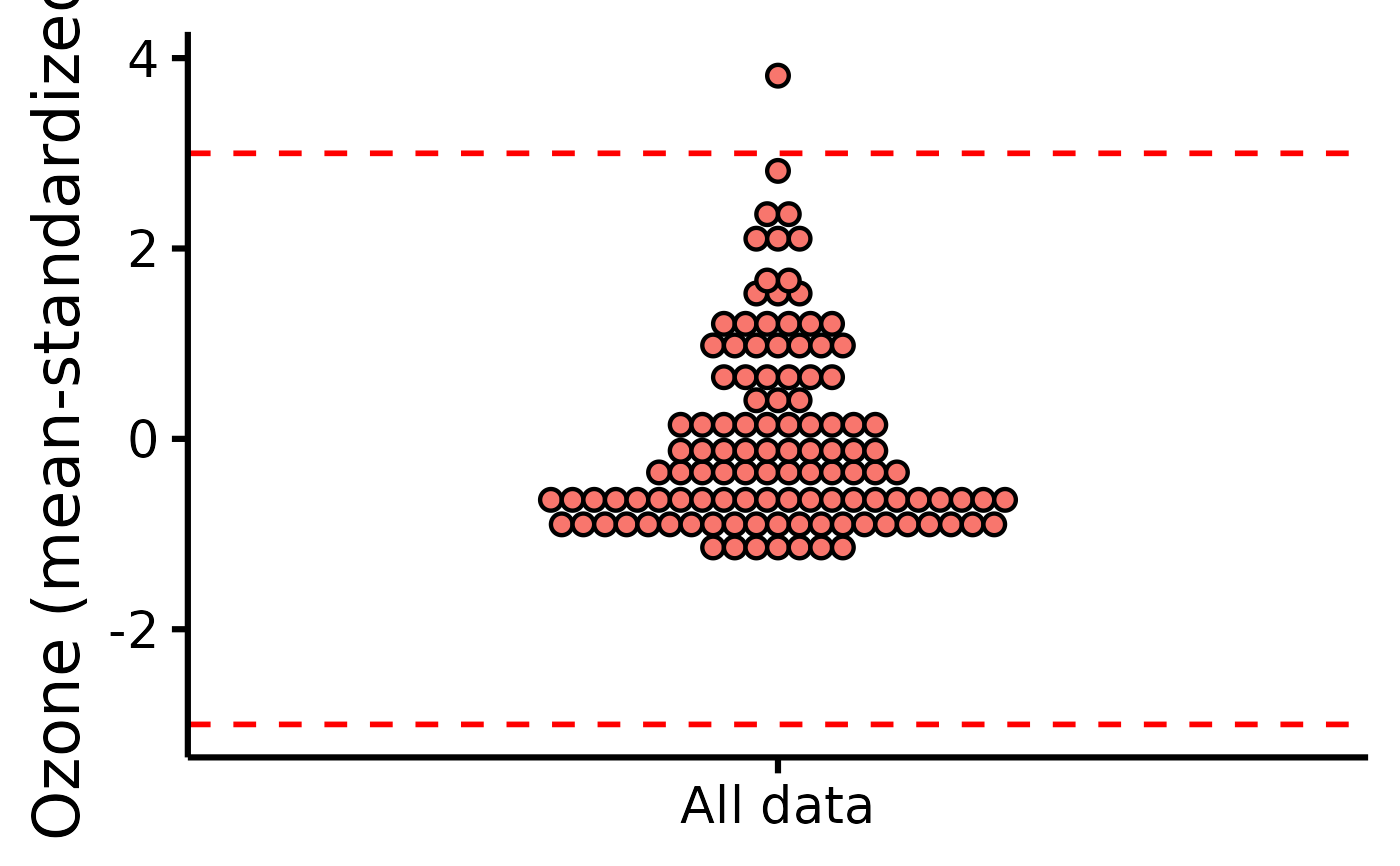Easily and visually check outliers through a dot plot with accompanying reference lines at +/- 3 MAD or SD. When providing a group, data are group-mean centered and standardized (based on MAD or SD); if no group is provided, data are simply standardized.

## Usage

plot_outliers(
data,
group = NULL,
response,
criteria = 3,
colours,
xlabels = NULL,
ytitle = NULL,
xtitle = NULL,
has.ylabels = TRUE,
has.xlabels = TRUE,
ymin,
ymax,
yby = 1,
...
)

## Arguments

data

The data frame.

group

The group by which to plot the variable.

response

The dependent variable to be plotted.

method

Method to identify outliers, either (e.g., 3) median absolute deviations ("mad", default) or standard deviations ("sd").

criteria

How many MADs (or standard deviations) to use as threshold (default is 3).

colours

Desired colours for the plot, if desired.

xlabels

The individual group labels on the x-axis.

ytitle

An optional y-axis label, if desired.

xtitle

An optional x-axis label, if desired.

has.ylabels

Logical, whether the x-axis should have labels or not.

has.xlabels

Logical, whether the y-axis should have labels or not.

ymin

The minimum score on the y-axis scale.

ymax

The maximum score on the y-axis scale.

yby

How much to increase on each "tick" on the y-axis scale.

...

Other arguments passed to ggplot2::geom_dotplot.

## Value

A dot plot of class ggplot, by group.

Other functions useful in assumption testing: Tutorial: https://rempsyc.remi-theriault.com/articles/assumptions

## Examples

# Make the basic plot
plot_outliers(
airquality,
group = "Month",
response = "Ozone"
)
#> Bin width defaults to 1/30 of the range of the data. Pick better value with
#> binwidth.
#> Warning: Removed 37 rows containing missing values (stat_bindot()).plot_outliers(
airquality,
response = "Ozone",
method = "sd"
)
#> Bin width defaults to 1/30 of the range of the data. Pick better value with
#> binwidth.
#> Warning: Removed 37 rows containing missing values (stat_bindot()).